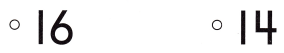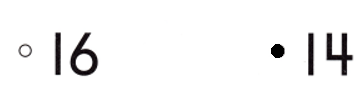# Texas Go Math Kindergarten Module 8 Assessment Answer Key

Refer to our Texas Go Math Kindergarten Answer Key Pdf to score good marks in the exams. Test yourself by practicing the problems from Texas Go Math Kindergarten Module 8 Assessment Answer Key.

## Texas Go Math Kindergarten Module 8 Assessment Answer Key

Concepts and Skills

DIRECTIONS: 1. Draw counters to show 16. Write the number of counters you drew. Write the number of counters in all. (TEKS K.2.B)

Question 1.Explanation:
Number of counters = 10 + 6 = 16 or Sixteen.

DIRECTIONS: 2-3. Write the number. (TEKS K.2.B) 4. Begin with 20. Write the number as you count backward from 20. (TEKS K.2.C) 5. Choose the correct answer. Which number is one less than 15? (TEKS K.2.F)

Question 2.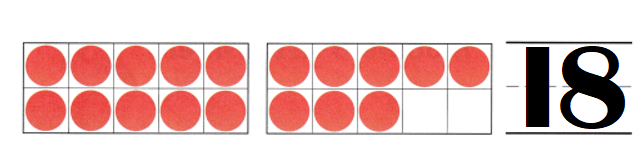Explanation:
Number of counters = 10 + 8 = 18 or Eighteen.

Question 3.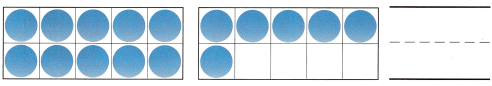Explanation:
Number of counters = 10 + 6 = Sixteen.

Question 4.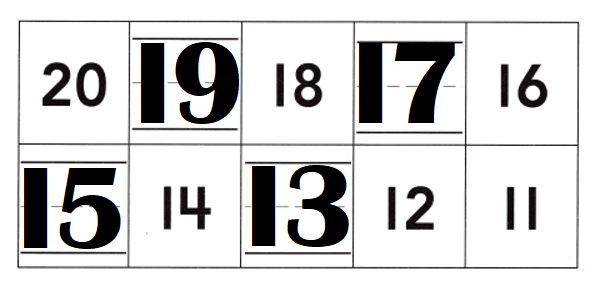Explanation:
Numbers backward from 20 : 20, 19, 18, 17, 16,15, 14,13, 12, 11.

Question 5.
Texas Test Prep